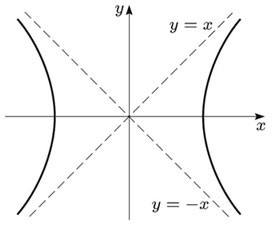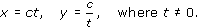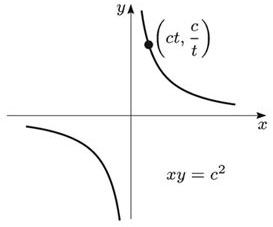Vectors and conics

This free course is available to start right now. Review the full course description and key learning outcomes and create an account and enrol if you want a free statement of participation.

Free course

4.7 Rectangular hyperbola (e = √2)

If the eccentricity e of a hyperbola is equal to √2, then e2 = 2 and b = a. Then the asymptotes of the hyperbola have equations y = ±x, so they are at right angles. A hyperbola whose asymptotes are at right angles is called a rectangular hyperbola.Then, if we use the asymptotes as new x- and y-axes (instead of the original x- and y-axes), the equation of the hyperbola can be written in the form xy = c2, for some positive number c. (We omit the details.)

The rectangular hyperbola with equation xy = c2 has the origin as its centre, and the x- and y-axes as its asymptotes. It can be described by the parametric equationsM208_1